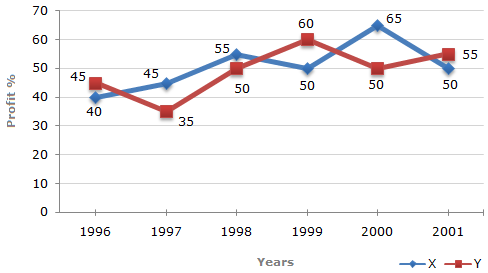### Line Chart - 3

The following line graph gives the percent profit earned by two Companies X and Y during the period 1996 - 2001.

Percentage profit earned by Two Companies X and Y over the Given Years

 %Profit = Income - Expenditure x 100 Expenditure1.  If the expenditure of Company Y in 1997 was ₹ 220 crores, what was its income in 1997 ?

 A. ₹ 312 crores B. ₹ 297 crores C. ₹ 283 crores D. ₹ 275 crores E. ₹ 261 crores
2.  The incomes of two Companies X and Y in 2000 were in the ratio of 3:4 respectively. What was the respective ratio of their expenditures in 2000 ?

 A. 7 : 22 B. 14 : 19 C. 15 : 22 D. 27 : 35 E. 33 : 40
3.  If the expenditures of Company X and Y in 1996 were equal and the total income of the two Companies in 1996 was ₹ 342 crores, what was the total profit of the two Companies together in 1996 ? (Profit = Income - Expenditure)

 A. ₹ 240 crores B. ₹ 171 crores C. ₹ 120 crores D. ₹ 102 crores E. None of these
4.  If the incomes of two Companies were equal in 1999, then what was the ratio of expenditure of Company X to that of Company Y in 1999 ?

 A. 6 : 5 B. 5 : 6 C. 11 : 6 D. 16 : 15 E. 15 : 16
5.  The expenditure of Company X in the year 1998 was ₹ 200 crores and the income of company X in 1998 was the same as its expenditure in 2001. The income of Company X in 2001 was ?

 A. ₹ 465 crores B. ₹ 385 crores C. ₹ 335 crores D. ₹ 295 crores E. ₹ 255 crores